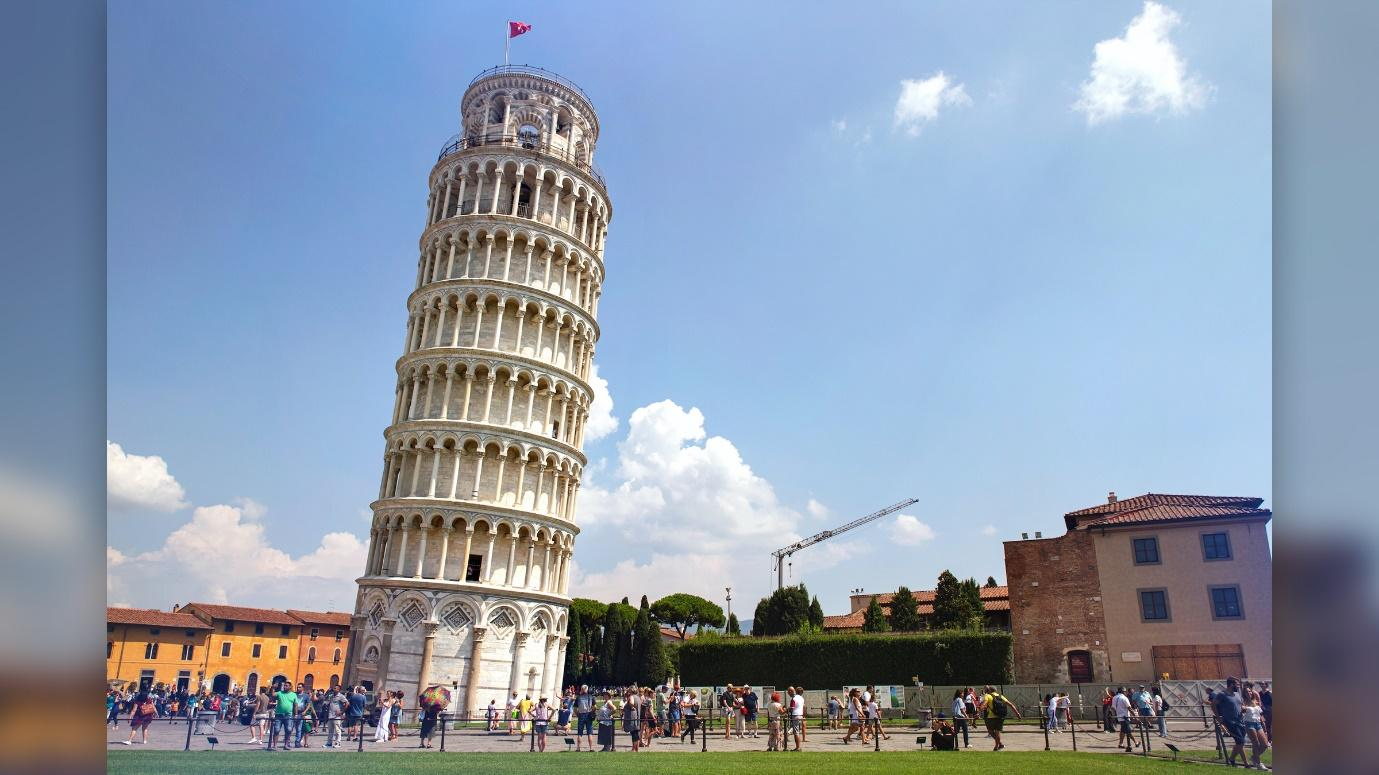12/8/2022

## Why Is Math Important?

By Jake Connell#### 1. Learn a language

Many people often don’t consider math as a creative subject, the way they do with language subjects. But, math is still a language. Unlike a spoken language such as French, English, or Spanish, however, a mathematical message is objective, precise, and has no ambiguity. Nowhere is this more clear than in computer programming, where you can ‘speak’ to a computer and tell it what to do through code. A strong understanding of linear algebra, Boolean algebra, calculus, and statistics are all extremely useful for coding and programming.

#### 2. Develop analytical thinking skills

All math problems require students to identify the problem and any important information that will be useful to solve the problem. These two things are large components of critical thinking – a skill that is important for pretty much everything.

#### 3. Feed unlimited people

Ever wonder how recipes are made? A bit of trial-and-error is expected, but math is always needed at some point! Once a recipe is produced for a certain number of people, mathematical ratios can be used to feed any number of people!

#### 4. Build things

A strong understanding of geometry, calculus, trigonometry, and statistics are required to create a building. Accurate and precise math ensures that the building you live in maintains its structural integrity for many years. If no one understood math, how could anything be built?

#### 5. Support a career

Ultimately, every career path has some level of math involved in it. Some level of arithmetic will be needed – the basic operations at the heart of mathematics. This includes, but is not limited to, invoices, calculating pay increases, taking measurements, and determining the cost of materials needed to complete a job.

#### 6. Become one with nature

Math appears naturally in many places. If we didn’t understand math, we would not be able to make sense of many things we see in nature. Some examples of math at work in nature include:

• Fibonacci sequences - spirals in nautilus shells, pine cone, petals on flowers
• Fractals (a mathematical shape that looks the same if you zoomed way in or way out) - found in tree branches, neurons in the brain, and coastlines

Fractals on a Tree Branch

Fibonacci Sequence in a Nautilus Shell

Want to share any thoughts or learn more about Renota? You can email us directly at info@Renota.app